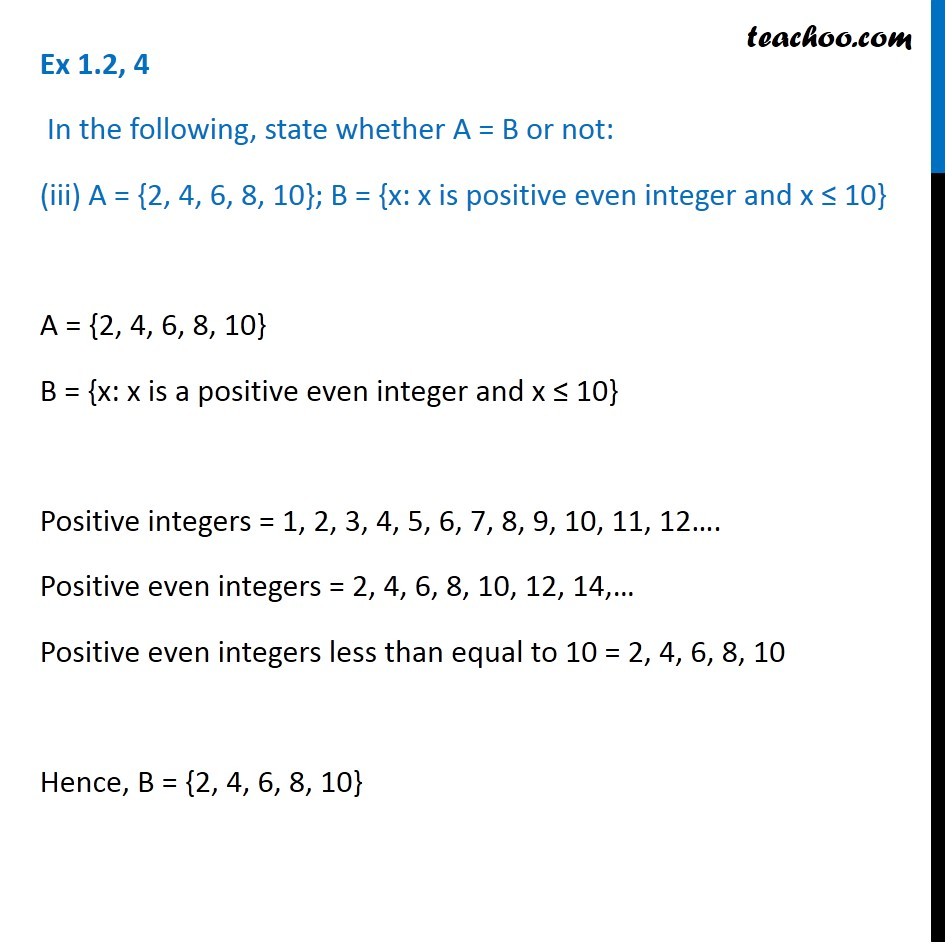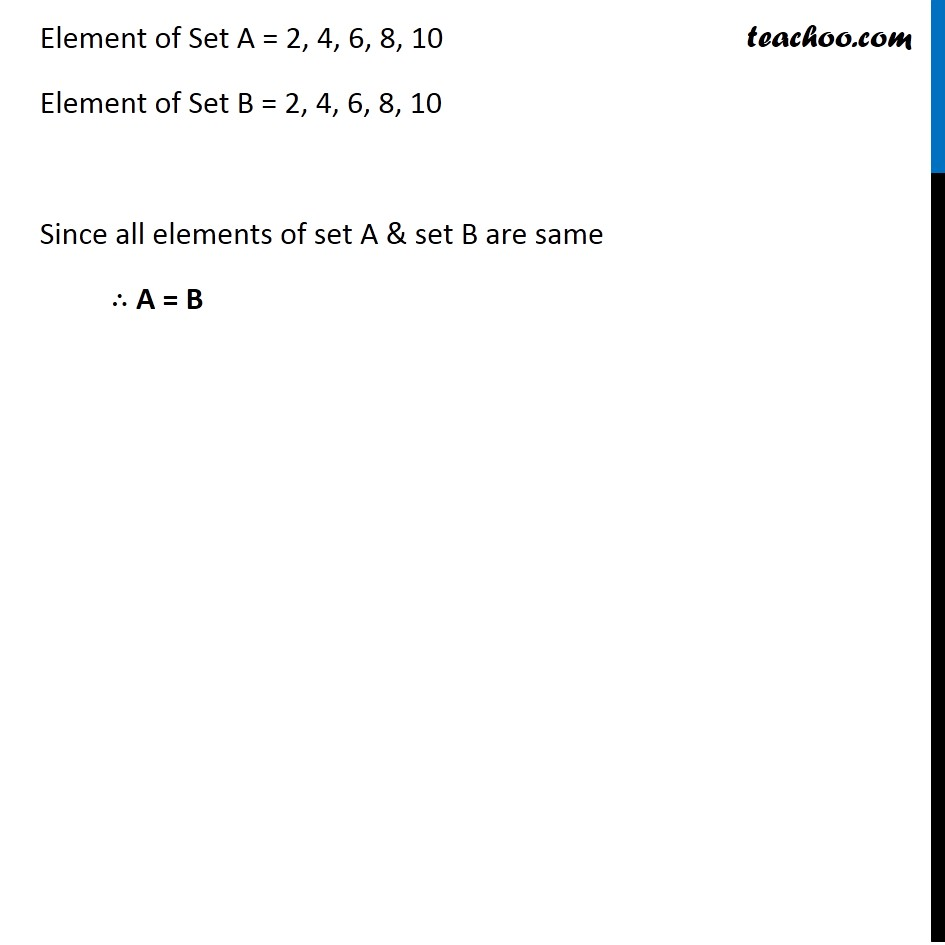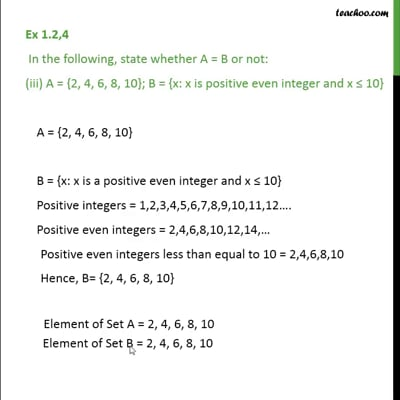Ex 1.2

Chapter 1 Class 11 Sets
Serial order wiseThis video is only available for Teachoo black users

Maths Crash Course - Live lectures + all videos + Real time Doubt solving!

### Transcript

Ex 1.2, 4 In the following, state whether A = B or not: (iii) A = {2, 4, 6, 8, 10}; B = {x: x is positive even integer and x ≤ 10} A = {2, 4, 6, 8, 10} B = {x: x is a positive even integer and x ≤ 10} Positive integers = 1, 2, 3, 4, 5, 6, 7, 8, 9, 10, 11, 12…. Positive even integers = 2, 4, 6, 8, 10, 12, 14,… Positive even integers less than equal to 10 = 2, 4, 6, 8, 10 Hence, B = {2, 4, 6, 8, 10} Element of Set A = 2, 4, 6, 8, 10 Element of Set B = 2, 4, 6, 8, 10 Since all elements of set A & set B are same ∴ A = B